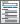Home  /  Products  /  Features  /  Other statistical methods

## Other statistical methods

Cronbach’s alpha• Interitem correlations or covariances
• Generate summative scale
• Automatically reverse sense of variables

Kappa measure of interrater agreement• Two unique raters
• Weights for weighting disagreements
• Nonunique raters, variables record ratings for each rater
• Nonunique raters, variables record frequency of ratings

Stepwise regression• Linear
• Beta
• Competing risks
• Complementary log-log
• Cox
• GLM
• Interval
• Interval-censored parametric survival
• Logistic
• Conditional logistic
• Negative binomial
• Ordered logit
• Ordered probit
• Poisson
• Probit
• Quantile
• Skewed logistic
• Tobit
• Exponential, Weibull, Gompertz, lognormal, loglogistic, generalized gamma parametric survival

Nested model statisticsKernel-density estimation• Eight different kernels
• Control band width
• Overlay normal density or Student's t density

Box–Cox transformPower transforms• Search for power transform that converts a variable into a normally distributed variable
• Graphical display of a power-transformed variable

Orthogonal polynomials• Orthogonalize variables using modified Gram–Schmidt procedures
• Compute orthogonal polynomial for a variable

Tests of normality

Drawing samples from multivariate normal distribution• Default is orthogonal data
• May specify desired means and covariance or correlation matrix
• Singular covariance matrix is permitted
• Set random-number seed to ensure reproducibility

Creating datasets with specified correlation structure• Add variables to existing dataset or create new dataset
• Singular covariance or correlation structures are permitted
• Set random-number seed to ensure reproducibility

Collecting statistics into a dataset• Collection from any command
• Collection of results for each group or subgroup of observations
• Collection from community-contributed or “official” commands